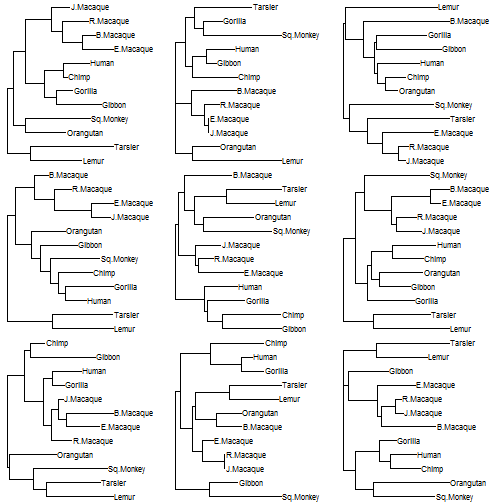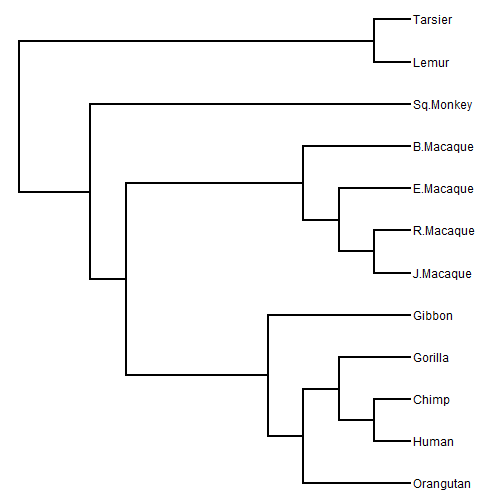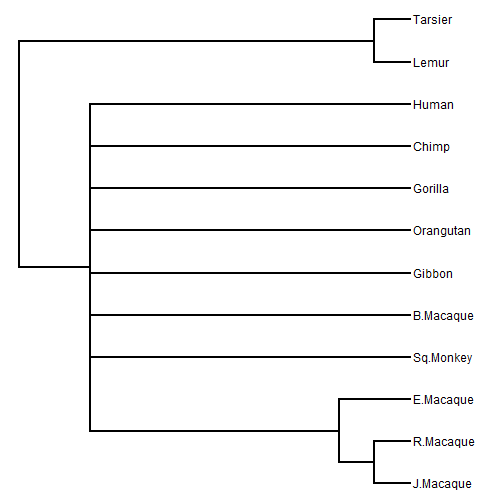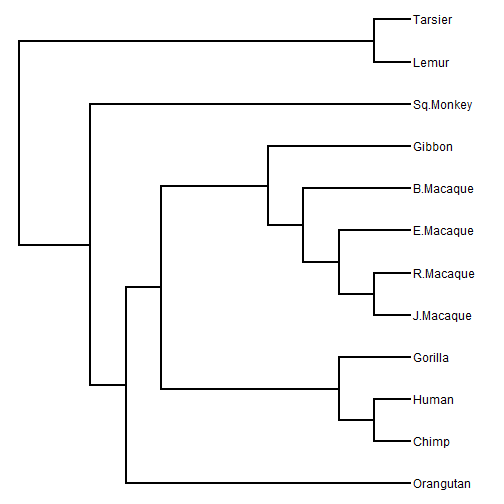## Thursday, April 7, 2016

### Consensus methods and computing the "average" of a set of phylogenies from a sample of trees

Since adding in some consensus approaches for the `"multiSimmap"` S3 `summary` method I have become interested in consensus and average trees. The idea here is to compute the average tree or consensus tree of a set of trees sampled from some distribution - not to be confused with methods designed to reconcile incompatible gene trees (which could be genuinely incongruent due to ILS or HGT), nor with super-tree methods designed to combine phylogenetic information for different subsets of taxa.

Most consensus methods tally the frequency in the sample with which each clade or bipartition is encountered. This approach is what is generally referred to as p-rule consensus tree estimation which can (sensibly) range from `p=0.5` “majority-rule” consensus to strict (`p=1`) consensus. Alternative, methods such as the maximum-clade-credibility (MCC) method tallies the support of clades across the entire sample, and then selects (from the sample) the tree with the highest overall clade support (& thus highest posterior probability).

A different approach is embodied by median tree methods in which the objective is to minimize distance to other trees, usually by computing distances (by some criterion) between all trees in the set, and then choosing (once again, from the set) the tree with the lowest summed or sum-of-squared distances to other trees. Of course, there is no theoretical difficulty with picking instead a tree not in the set that has minimum distance (or minimum sum-of-squares distance) to all trees in the set. This is what I will refer to as the average tree.

Now, I have no idea whether the average tree from a set of trees sampled from a common distribution (say, sampled from a Bayesian posterior probability distribution using MCMC) has good properties or bad properties, but it nonetheless seems like it might be interesting to compute - and I have now added a set of new functions to the phytools package (see update here) that perform this functionality.

To summarize, `averageTree` tries to find the “average” tree (the tree with minimum sum of squared distances to all other trees, by various distance criteria), `minTreeDist` computes (for distance measures that take branch lengths into account) the branch lengths that minimize the sum of squares distances between a reference tree and a set of trees, and `ls.consensus` computes the least-squares consensus tree from the mean patristic distance matrix of a set of trees. This final function is related to average trees in a way that will be discussed in a future post.

Right now these functions can be a little bit slow. This is because each time the tree is changed, the distances to all the other trees must be recomputed. In addition, the optimization method in `averageTree` uses only nearest-neighbor-interchanges (NNIs), which are not particularly aggressive and thus may not encounter the best tree by the stated criterion overall (although the chances of this are improved if we start with a pretty good tree).

In the following I have a simple demo using a sample of 100 trees of primates, each with 12 tips. These were generated by jackknife resampling with very few nucleotides the `primates` dataset packaged with our Rphylip package.

``````library(phytools)
trees
``````
``````## 100 phylogenetic trees
``````

Though these trees all come from the same distribution, most are quite bad. So, for instance, if we plot these (re-rooting each one at it's midpoint midpoints so that they look sensible), we'll quickly see that many reflect the fairly well-known underlying relationships of these taxa quite poorly:

``````par(mfrow=c(10,10))
nulo<-lapply(lapply(trees,midpoint),plotTree,fsize=0.5,lwd=1)
``````Or, if we were to pick some at random instead:

``````par(mfrow=c(3,3))
nulo<-lapply(lapply(trees[sample(1:100,9)],midpoint),plotTree,lwd=1)
``````Now, I'll just quickly demonstrate one of the criteria for which we might pick an “average” tree, and that is using the well-known Robinson-Foulds distance:

``````rf.tree<-averageTree(trees,method="symmetric.difference")
``````
``````##
##   Function is attempting to find the phylogeny with
##   minimum distance to all trees in set under the
##   "symmetric difference" criterion...
``````
``````##   Best SS so far = 17240
``````
``````##   Best SS so far = 15792
``````
``````##   Best SS so far = 14512
``````
``````##   Best SS so far = 13804
``````
``````##   Best SS so far = 11796
``````
``````##   Best SS so far = 11348
##   Best SS so far = 11348
``````
``````##
##   Solution found after 7 set of nearest neighbor interchanges.
``````

Note that sometimes this does not converge on the best tree (remember, optimization is performed using NNIs), so it could be useful to provide a pretty good `start` tree, or to run multiple times with different values for `start` to ensure convergence.

This tree is probably pretty good. The tree will be unrooted & lacks branch lengths as all we have optimized is topological distance, but it generally reflects the topological relations that we think probably uderlies this group of species:

``````plotTree(root(rf.tree,outgroup=c("Tarsier","Lemur"),resolve.root=TRUE))
``````We can compare this to a majority-rule consensus tree - but I bet you can guess what will happen:

``````plotTree(root(consensus(trees,p=0.5),outgroup=c("Tarsier","Lemur"),
resolve.root=TRUE))
``````It's clear that the resolution of the consensus tree is bad, even though the average tree is reasonably close (or possibly equal) to the true tree.

This is not meant to be a critique of consensus tree methods, and if it were it would be bad one as this sample of trees does not (say) correspond to the sample one would obtain using Bayesian MCMC; however it is interesting to see that the average and consensus tree approaches can yield substantively different outcomes.

Finally, we can compare this to the “median” tree which is the sampled tree with the lowest (squared) distance to all the trees in the sample.

Here this is calculable as follows:

``````ss.d<-colSums(as.matrix(RF.dist(trees))^2)
ii<-which(ss.d==min(ss.d))
ss.d[ii]
``````
``````##    82
## 12544
``````
``````plotTree(compute.brlen(root(trees[[ii]],outgroup=c("Tarsier","Lemur"),
resolve.root=TRUE)))
``````We see here that though the average tree is good, the best tree in the sample evaluated on the same criterion - distance to the other trees - is not particularly good!

There are other criteria under which we might compute an “average tree,” and some of these include edge lengths. In these cases, which I'll discuss in subsequent posts, we need to optimize not only the topology but the branch lengths of the average tree.

This latest version of phytools can be installed from GitHub using the devtools package as follows:

``````library(devtools)
install_github("liamrevell/phytools")
``````

The trees for this exercise were generated as follows:

``````library(Rphylip)
data(primates)
bs<-function(x){
x<-as.matrix(x)
x[,sample(1:ncol(x),20,replace=TRUE)]
}
bs.primates<-replicate(100,bs(primates),simplify=FALSE)
foo<-function(x) NJ(dist.dna(x,model="raw"))
trees<-lapply(bs.primates,foo)
class(trees)<-"multiPhylo"
``````

Finally, I'll add that this is part of what I hope will be a talk or publication exploring the effects of consensus methods on PCMs, and that this is being conducted in collaboration with my postdoc Klaus Schliep, with the contribution of some ideas by Cristián Hernández Ulloa.

1.I would like to tree averageTree function but its not in my version of phytools!

1.See the post above:

"This latest version of phytools can be installed from GitHub using the devtools package as follows:

library(devtools)
install_github("liamrevell/phytools")

"

2.Novice to phylogenetics and just playing around with some assemblages at the moment and found this post helpful. Thanks!

3.This comment has been removed by the author.

Note: due to the very large amount of spam, all comments are now automatically submitted for moderation.